# Question Solved1 AnswerQuestion 22 B W 4 X Z IV Y A rectangular loop of wire consisting of wire segments W X, Y, and Z is in a region of uniform magnetic field that is directed to the left, as shown. There is a counterclockwise current I flowing through the loop. Which selection best describes the rotation, if any, of the loop and the magnetic forces on the segments of the loop? А There will be no rotation of the loop because there is no magnetic force on the loop. The loop is in the plane of the magnetic field, and there must be an angle between the loop and the field for there to be a magnetic force on the loop. B There will be no rotation of the loop because the magnetic forces on the loop are all perpendicular to the segments and directed outward. These forces tend to stretch the loop but not rotate it. с The loop will tend to rotate with segment W moving out of the plane of the page, because there is a magnetic force on segments W and Y but not on X and Z D The loop will tend to rotate with segment X moving into the plane of the page, because there is a magnetic force on segments X and Z but not on W and Y E The loop will not rotate, but will spin in the plane of the page because the magnetic forces are all in the plane of the page. Question 24 0 L -B 1 W In an experiment, students place a rectangular conducting loop of length L and width w in a uniform magnetic field of magnitude B, as shown. A power supply (not shown) is used to generate a current of magnitude I in the loop, and a force sensor (not shown) is used to measure the magnetic force F on the loop. The students then calculate the torque on the loop. Which of the following correctly explains how a source of error could affect the results of the experiment? A If the measured current is less than the actual current, then the actual torque exerted on the loop will be greater than the calculated torque. B If the measured magnetic field strength is greater than the actual magnetic field strength, then the actual torque exerted on the loop will be greater than the calculated torque. с If the measured force is greater than the actual force, then the actual torque exerted on the loop will be greater than the calculated torque. D If the measured length is greater than the actual length, then the actual torque exerted on the loop will be greater than the calculated torque. E If the measured width is greater than the actual width, then the actual torque exerted on the loop will be greater than the calculated torque. Question 25 0 - - 12 / •P Wire 1 Wire 2 Wires 1 and 2 are a distance d apart and each carries a current I toward the bottom of the page, as shown. Point Pis a distance to the right of wire 2. What is the magnitude of the magnetic field at point P? А Zero B с Po! D 3xd Question 27 a 1 Wire 1 Wire 2 Long, straight, parallel wires 1 and 2 carry current I in opposite directions, as shown. Which of the following correctly describes the magnetic fields from the wires in the region between the wires and provide evidence to support this claim? The magnetic fields are in opposite directions, because the currents are in opposite directions. B The magnetic fields are in opposite directions, because the region is on opposite sides of the wires: to the right of wire 1 and to the left of wire 2. The magnetic fields are in the same directions, because both wires are long and straight. D The magnetic fields are in the same directions, because the currents have the same magnitude. The magnetic fields are in the same directions, because the currents are in opposite directions and are on opposite sides of the wires: to the right of wire 1 and to the left of wire 2.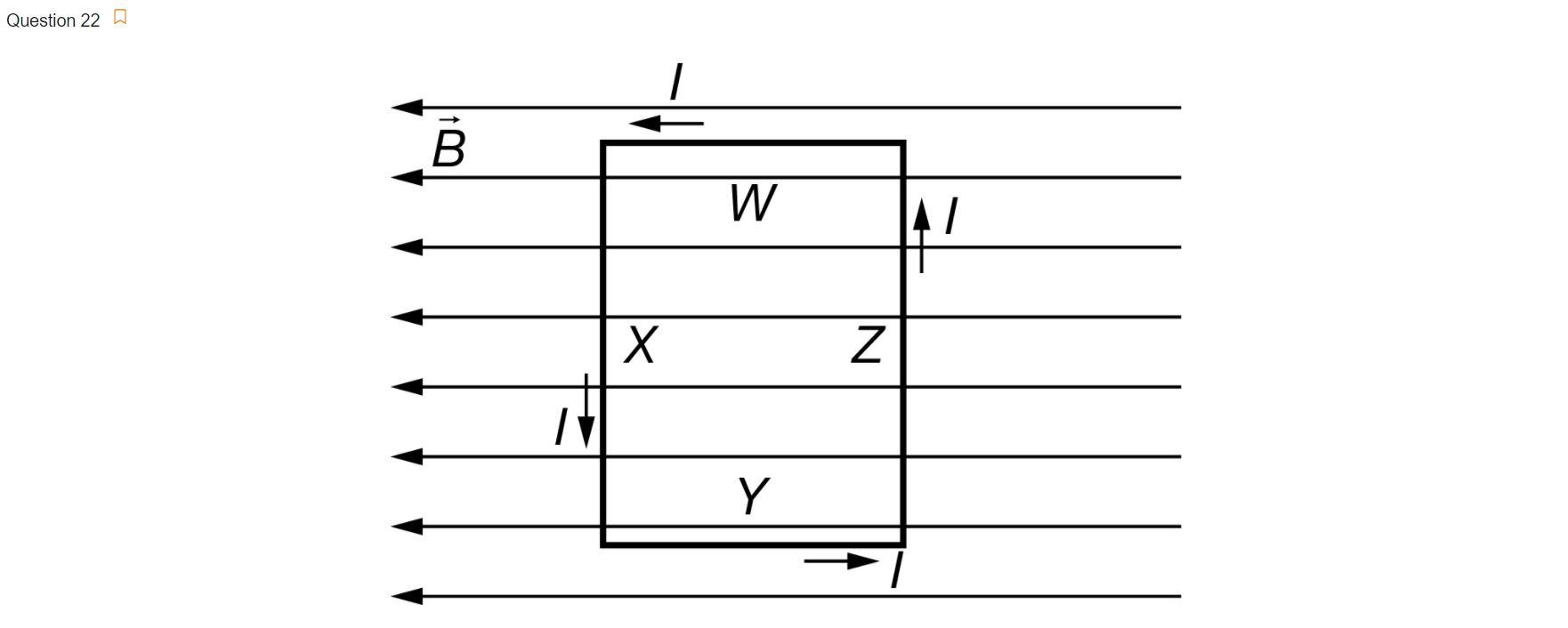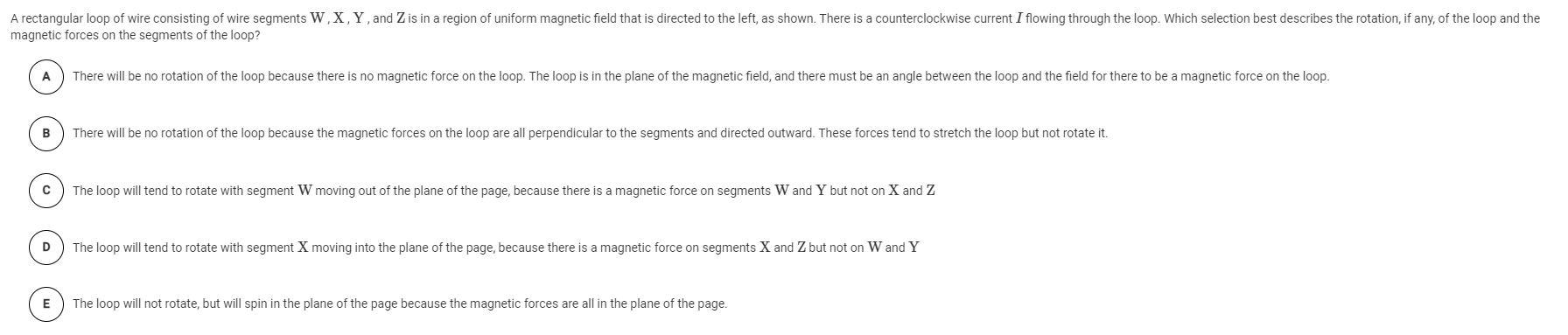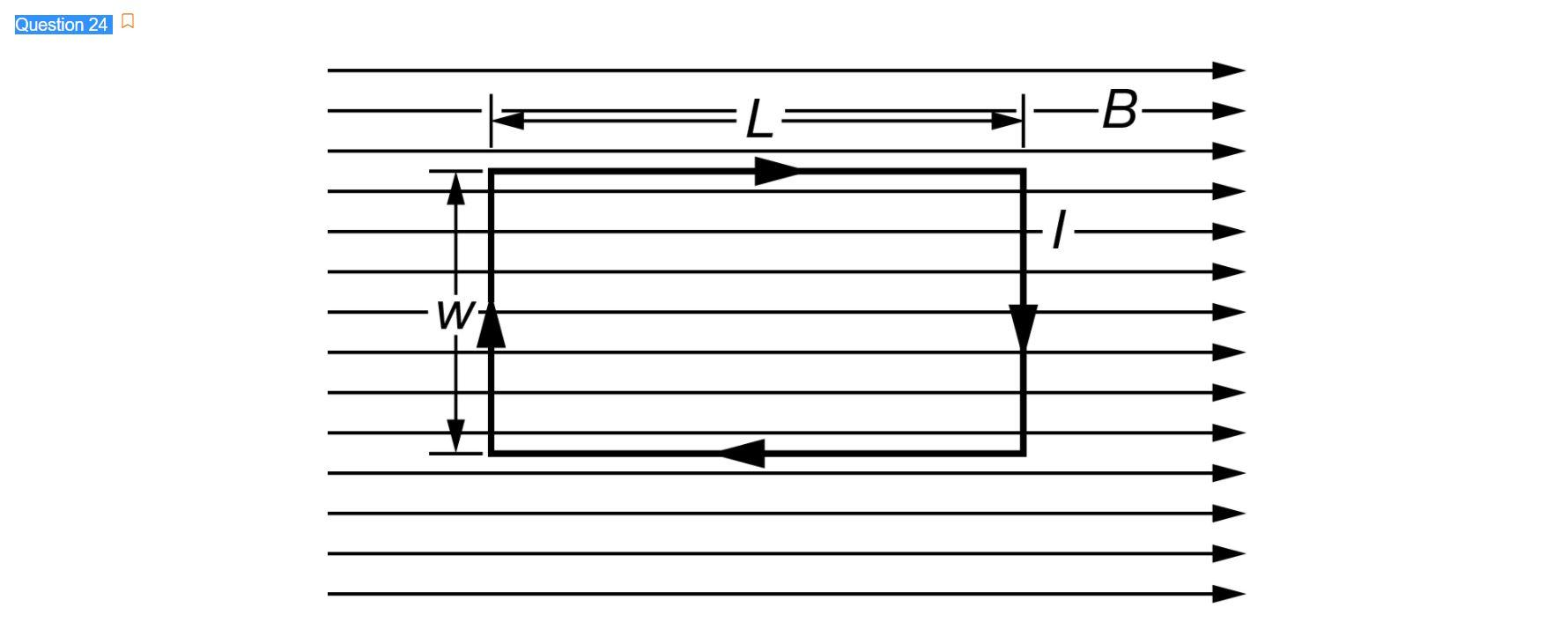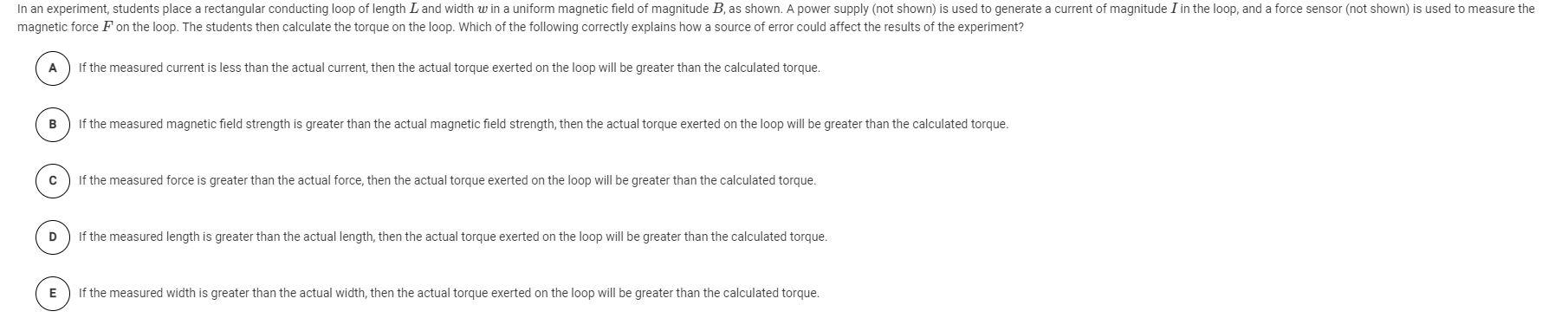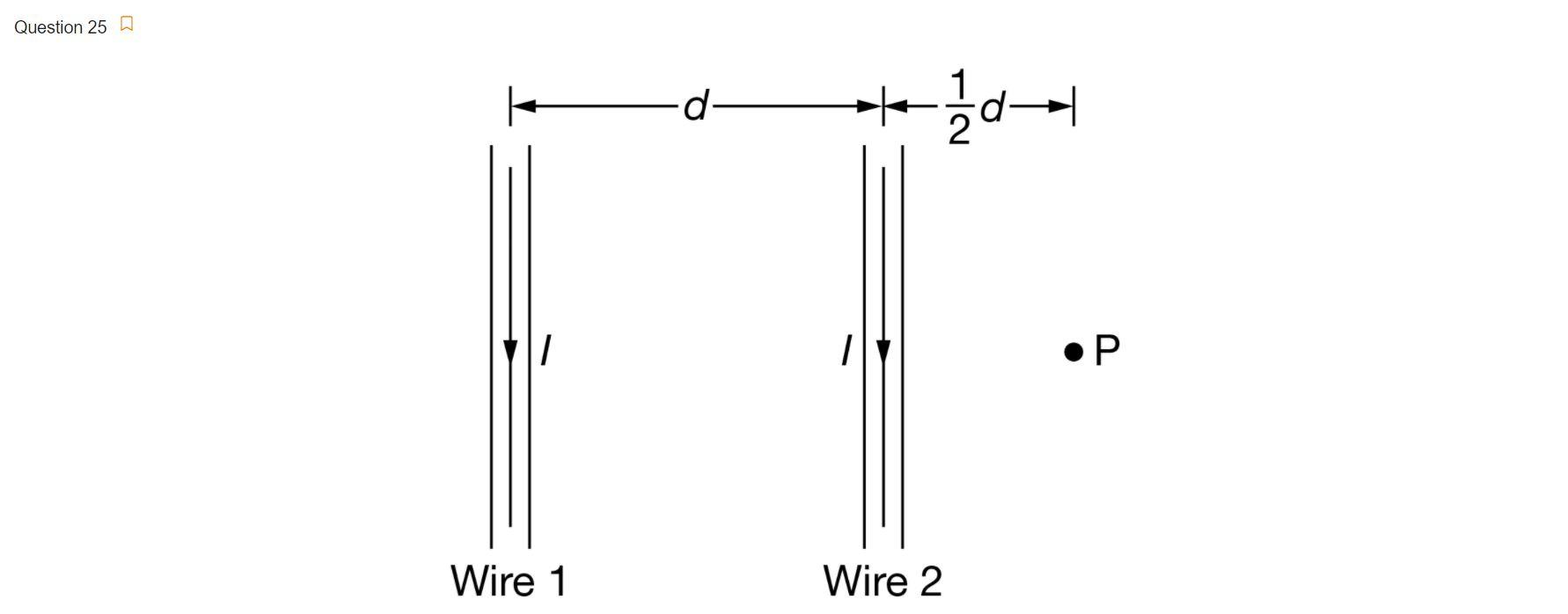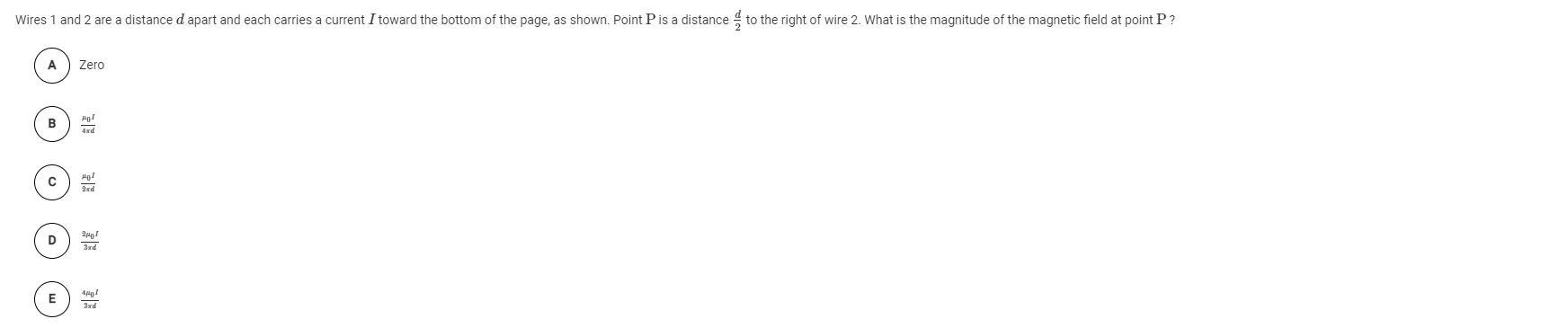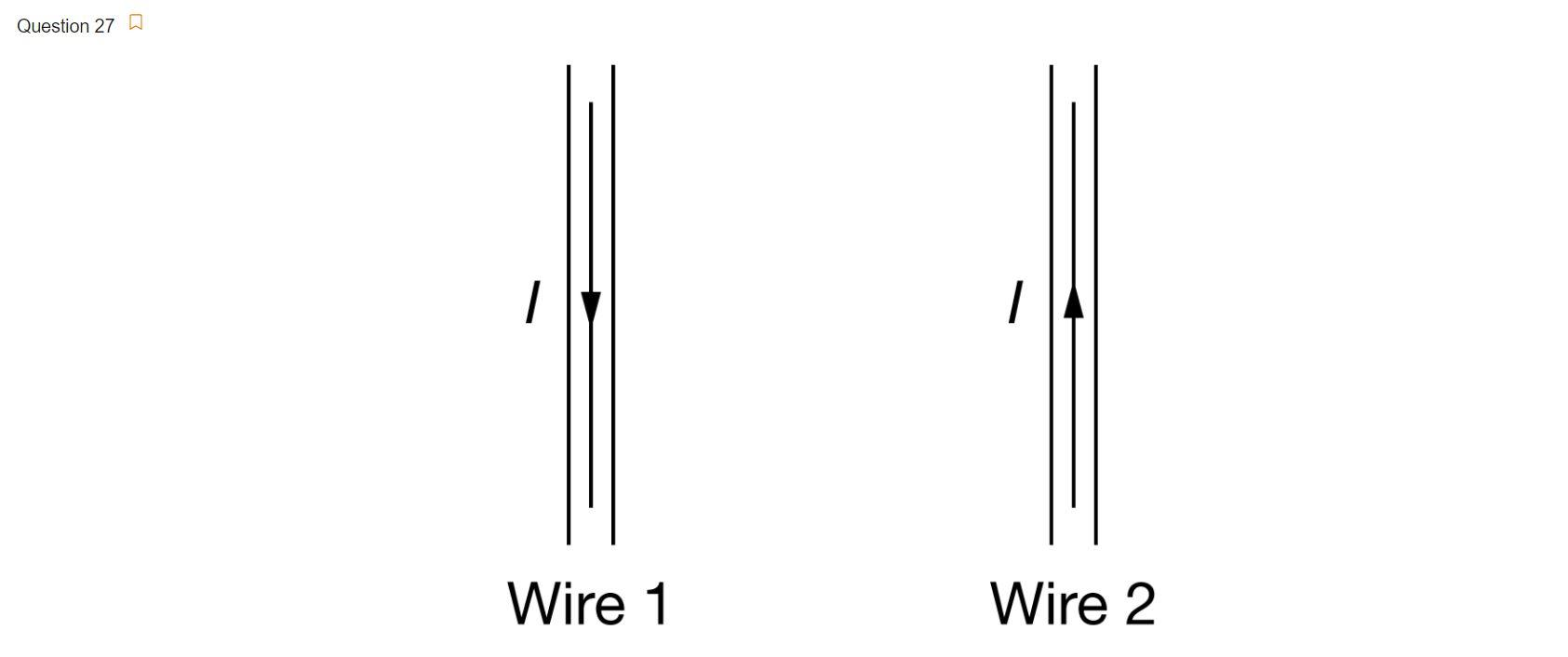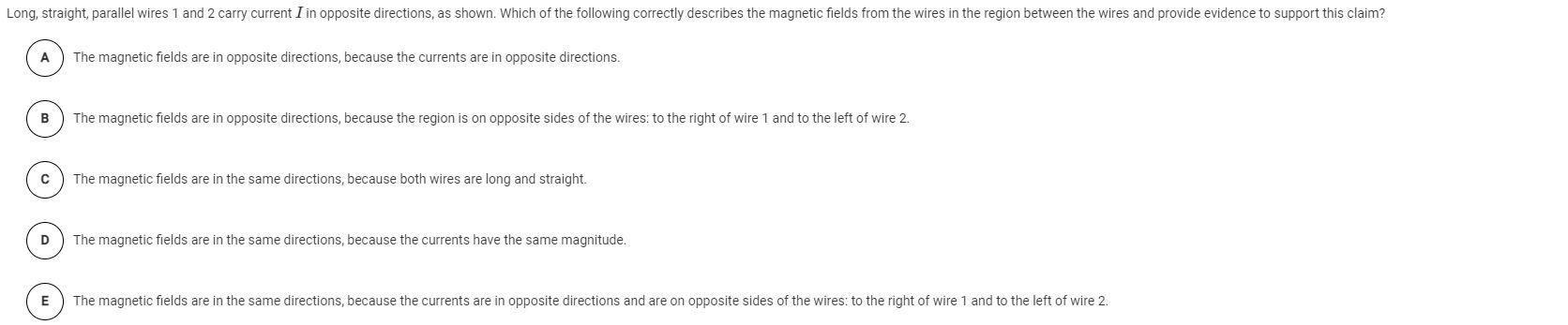Transcribed Image Text: Question 22 B W 4 X Z IV Y A rectangular loop of wire consisting of wire segments W X, Y, and Z is in a region of uniform magnetic field that is directed to the left, as shown. There is a counterclockwise current I flowing through the loop. Which selection best describes the rotation, if any, of the loop and the magnetic forces on the segments of the loop? А There will be no rotation of the loop because there is no magnetic force on the loop. The loop is in the plane of the magnetic field, and there must be an angle between the loop and the field for there to be a magnetic force on the loop. B There will be no rotation of the loop because the magnetic forces on the loop are all perpendicular to the segments and directed outward. These forces tend to stretch the loop but not rotate it. с The loop will tend to rotate with segment W moving out of the plane of the page, because there is a magnetic force on segments W and Y but not on X and Z D The loop will tend to rotate with segment X moving into the plane of the page, because there is a magnetic force on segments X and Z but not on W and Y E The loop will not rotate, but will spin in the plane of the page because the magnetic forces are all in the plane of the page. Question 24 0 L -B 1 W In an experiment, students place a rectangular conducting loop of length L and width w in a uniform magnetic field of magnitude B, as shown. A power supply (not shown) is used to generate a current of magnitude I in the loop, and a force sensor (not shown) is used to measure the magnetic force F on the loop. The students then calculate the torque on the loop. Which of the following correctly explains how a source of error could affect the results of the experiment? A If the measured current is less than the actual current, then the actual torque exerted on the loop will be greater than the calculated torque. B If the measured magnetic field strength is greater than the actual magnetic field strength, then the actual torque exerted on the loop will be greater than the calculated torque. с If the measured force is greater than the actual force, then the actual torque exerted on the loop will be greater than the calculated torque. D If the measured length is greater than the actual length, then the actual torque exerted on the loop will be greater than the calculated torque. E If the measured width is greater than the actual width, then the actual torque exerted on the loop will be greater than the calculated torque. Question 25 0 - - 12 / •P Wire 1 Wire 2 Wires 1 and 2 are a distance d apart and each carries a current I toward the bottom of the page, as shown. Point Pis a distance to the right of wire 2. What is the magnitude of the magnetic field at point P? А Zero B с Po! D 3xd Question 27 a 1 Wire 1 Wire 2 Long, straight, parallel wires 1 and 2 carry current I in opposite directions, as shown. Which of the following correctly describes the magnetic fields from the wires in the region between the wires and provide evidence to support this claim? The magnetic fields are in opposite directions, because the currents are in opposite directions. B The magnetic fields are in opposite directions, because the region is on opposite sides of the wires: to the right of wire 1 and to the left of wire 2. The magnetic fields are in the same directions, because both wires are long and straight. D The magnetic fields are in the same directions, because the currents have the same magnitude. The magnetic fields are in the same directions, because the currents are in opposite directions and are on opposite sides of the wires: to the right of wire 1 and to the left of wire 2.
More
Transcribed Image Text: Question 22 B W 4 X Z IV Y A rectangular loop of wire consisting of wire segments W X, Y, and Z is in a region of uniform magnetic field that is directed to the left, as shown. There is a counterclockwise current I flowing through the loop. Which selection best describes the rotation, if any, of the loop and the magnetic forces on the segments of the loop? А There will be no rotation of the loop because there is no magnetic force on the loop. The loop is in the plane of the magnetic field, and there must be an angle between the loop and the field for there to be a magnetic force on the loop. B There will be no rotation of the loop because the magnetic forces on the loop are all perpendicular to the segments and directed outward. These forces tend to stretch the loop but not rotate it. с The loop will tend to rotate with segment W moving out of the plane of the page, because there is a magnetic force on segments W and Y but not on X and Z D The loop will tend to rotate with segment X moving into the plane of the page, because there is a magnetic force on segments X and Z but not on W and Y E The loop will not rotate, but will spin in the plane of the page because the magnetic forces are all in the plane of the page. Question 24 0 L -B 1 W In an experiment, students place a rectangular conducting loop of length L and width w in a uniform magnetic field of magnitude B, as shown. A power supply (not shown) is used to generate a current of magnitude I in the loop, and a force sensor (not shown) is used to measure the magnetic force F on the loop. The students then calculate the torque on the loop. Which of the following correctly explains how a source of error could affect the results of the experiment? A If the measured current is less than the actual current, then the actual torque exerted on the loop will be greater than the calculated torque. B If the measured magnetic field strength is greater than the actual magnetic field strength, then the actual torque exerted on the loop will be greater than the calculated torque. с If the measured force is greater than the actual force, then the actual torque exerted on the loop will be greater than the calculated torque. D If the measured length is greater than the actual length, then the actual torque exerted on the loop will be greater than the calculated torque. E If the measured width is greater than the actual width, then the actual torque exerted on the loop will be greater than the calculated torque. Question 25 0 - - 12 / •P Wire 1 Wire 2 Wires 1 and 2 are a distance d apart and each carries a current I toward the bottom of the page, as shown. Point Pis a distance to the right of wire 2. What is the magnitude of the magnetic field at point P? А Zero B с Po! D 3xd Question 27 a 1 Wire 1 Wire 2 Long, straight, parallel wires 1 and 2 carry current I in opposite directions, as shown. Which of the following correctly describes the magnetic fields from the wires in the region between the wires and provide evidence to support this claim? The magnetic fields are in opposite directions, because the currents are in opposite directions. B The magnetic fields are in opposite directions, because the region is on opposite sides of the wires: to the right of wire 1 and to the left of wire 2. The magnetic fields are in the same directions, because both wires are long and straight. D The magnetic fields are in the same directions, because the currents have the same magnitude. The magnetic fields are in the same directions, because the currents are in opposite directions and are on opposite sides of the wires: to the right of wire 1 and to the left of wire 2.